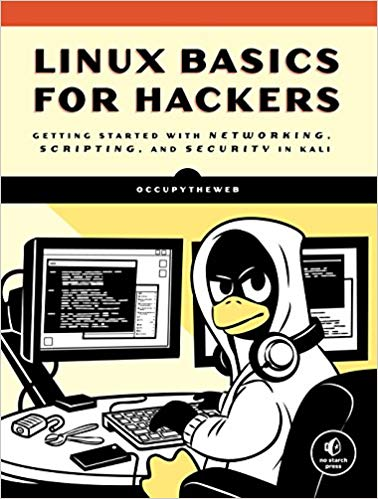# Linux Basics for Hacking and Hackers Latest Technics

Step 1: Check Permissions (Permits)

If we want to find permissions for a file, we can simply use the ls command with the -l switch.

Let’s use this command in the usr / share / wireshark / directory and see what it says about the files in it.If we look at each line, we can see a small amount of files, including whether it is a file or a directory, permissions in the file, the number of links, the owner of the file, the owner of the file group, the file size, when it is created or modified. And finally, the file name. Let’s examine each of these.

#### Detect a file or directory

The first character of each line tells us whether it is a file or a directory. If the line starts with d, it is a directory. If it starts with “-” it is a file.Next characters determine the file permissions. There are three sets of rwx that have permission to read, write, and execute files.

These characters determine whether there is a file read permission, whether the file can be edited or the file executed. Each set of rwx represents the owner’s license, group, and others.

(w)write

(x)execute

So, if we look at the second line for the file ABOUT.GPL …#### We see that it started this way:

````-rw-r--r--`

This means that it has a (-) file that owns the read (r) and write (w) permissions, but does not have permission to run (-).The next set of permissions represents the group. Here we can see that the group has read permission (r) but does not write (-) and execute (-).Finally, the last set of permissions is for everyone or others. We can see that others only have permission to read (r) the file ABOUT.GPL.```

#### Step 2: Change the permissions

Let’s imagine what we want, the group can edit and run the ABOUT.GPL file. Linux has a command named chmod that allows you to change the permissions of a file.

Of course, as long as we are the root user or the owner of the file. These licenses are shown by their binary equivalent in the operating system.

#### Numerical method

In numerical methods, for each license, is an equivalent number.

r w x

4 2 1 = 7

The sum of these three is equal to seven. When we want to file all the permissions (reading, editing, executing) into a file, we show it with a numeric equivalent of 7.

So, if we want the owner (7) and group (7) and others or all users (7) have all file permissions. We can show it like this:

777

Now let’s go back to our ABOUT.GPL file, remember that their permissions were rw-r-r- so we can quote it numerically:

r w – r – – r – –

4 2 0 4 0 0 4 0 0

This can be displayed with 644.

#### Change current permissions for ABOUT.GPL

Now, if we wanted to give the group the edit license (2) and run (1), we can use the chmod command to do this.

We need to add write permission (2) and run permission (1) to the file ABOUT.GPL. Like below:

``chmod 7 7 4 ABOUT.GPL``It says the owner has all three licenses (4 + 2 + 1 = 7), the group (4 + 2 + 1 = 7). And gives others permission to read (4 + 0 + 0 = 4).

When we run ls -l, we can see that the ABOUT.GPL permissions are as follows:

````r w x r w x r - -`

```

#### Step 3: Change the license with UGO

Although the numerical method is probably the most common way to change permissions on Linux, there is another way that some people are more comfortable with.

It is often referred to as UGO. UGO stands for U = User or Owner, G = Group and O = Others. UGO has three operators:So, if we want to get permission to write from the group that ABOUT.GPL belongs to. We write:

``chmod g-w ABOUT.GPL``This command says “Decide for group (g) (-) write permission (w) for the file ABOUT.GPL”

You can see that when I check the file permissions by typing ls -l, the ABOUT.GPL file does not have another write permission for the group.

If I want to run the user and the group at the same time, I can type:

````chmod u+x, g+x ABOUT.GPL`

```

This command says: “To add a user, run permissions, add to the group the run permission for the file ABOUT.GPL”

### Step 4: Give permission to run the new hacking tools

If we do not do this, we usually receive a message that does not have sufficient permission to run it.

In the screenshot below, we see that our 1newtool file does not have permission to run on anyone. We can give it a license with the following command.````chmod 766 1newtool````

As you already know, it gives us, the owner, all permissions including execution, and gives the group and anyone else permissions to read and write (4 + 2 = 6).

You can see in the image above that chmod is exactly what we want!

```
```